# Mathematical economics

Mathematical economics is the application of mathematical methods to represent theories and analyze problems in economics. Often, these applied methods are beyond simple geometry, and may include differential and integral calculus, difference and differential equations, matrix algebra, mathematical programming, or other computational methods. Proponents of this approach claim that it allows the formulation of theoretical relationships with rigor, generality, and simplicity. Mathematics allows economists to form meaningful, testable propositions about wide-ranging and complex subjects which could less easily be expressed informally. Further, the language of mathematics allows economists to make specific, positive claims about controversial or contentious subjects that would be impossible without mathematics. Much of economic theory is currently presented in terms of mathematical economic models, a set of stylized and simplified mathematical relationships asserted to clarify assumptions and implications. Broad applications include: * optimization problems as to goal equilibrium, whether of a household, business firm, or policy maker * static (or equilibrium) analysis in which the economic unit (such as a household) or economic system (such as a market or the economy) is modeled as not changing * comparative statics as to a change from one equilibrium to another induced by a change in one or more factors * dynamic analysis, tracing changes in an economic system over time, for example from economic growth. Formal economic modeling began in the 19th century with the use of differential calculus to represent and explain economic behavior, such as utility maximization, an early economic application of mathematical optimization. Economics became more mathematical as a discipline throughout the first half of the 20th century, but introduction of new and generalized techniques in the period around the Second World War, as in game theory, would greatly broaden the use of mathematical formulations in economics. This rapid systematizing of economics alarmed critics of the discipline as well as some noted economists. John Maynard Keynes, Robert Heilbroner, Friedrich Hayek and others have criticized the broad use of mathematical models for human behavior, arguing that some human choices are irreducible to mathematics. (Wikipedia).Introduction to Economics

What is economics? Yes, of course it has to do with money. But beyond the concepts that we might immediately think of, like taxes, gross domestic product, and the stock market, which belong to macroeconomics, we must also understand microeconomics. Deconstructing the personal decisions we

From playlist Economics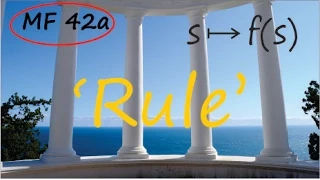The problem with `functions' | Arithmetic and Geometry Math Foundations 42a

[First of two parts] Here we address a core logical problem with modern mathematics--the usual definition of a `function' does not contain precise enough bounds on the nature of the rules or procedures (or computer programs) allowed. Here we discuss the difficulty in the context of funct

From playlist Math Foundations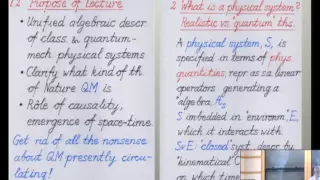Quantum Mechanics -- a Primer for Mathematicians

Juerg Frohlich ETH Zurich; Member, School of Mathematics, IAS December 3, 2012 A general algebraic formalism for the mathematical modeling of physical systems is sketched. This formalism is sufficiently general to encompass classical and quantum-mechanical models. It is then explained in w

From playlist Mathematics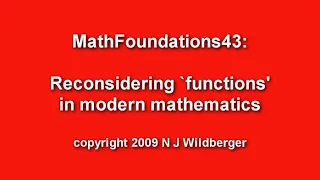Reconsidering `functions' in modern mathematics | Arithmetic and Geometry Math Foundations 43

The general notion of `function' does not work in mathematics, just as the general notions of `number' or `sequence' don't work. This video explains the distinction between `closed' and `open' systems, and suggests that mathematical definitions should respect the open aspect of mathemat

From playlist Math FoundationsUsing Algebra and Geometry in the Real World

You hear terms like “algebra” and “geometry” and these theories we memorized in high school start to dance a jig in our heads – a jig many of us weren’t overly interested in! But the past decade has seen an explosion of applications of algebra, geometry, and topology to the real world, lik

From playlist What is math used for?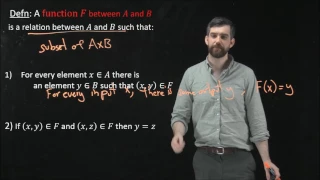Formal Definition of a Function using the Cartesian Product

Learning Objectives: In this video we give a formal definition of a function, one of the most foundation concepts in mathematics. We build this definition out of set theory. **************************************************** YOUR TURN! Learning math requires more than just watching vid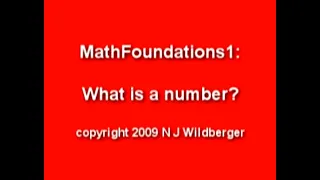What is a number? | Arithmetic and Geometry Math Foundations 1 | N J Wildberger

The first of a series that will discuss foundations of mathematics. Contains a general introduction to the series, and then the beginnings of arithmetic with natural numbers. This series will methodically develop a lot of basic mathematics, starting with arithmetic, then geometry, then alg

From playlist Math Foundations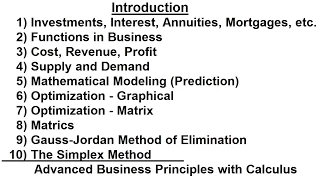Business Math (1 of 1) Introduction

Visit http://ilectureonline.com for more math and science lectures! In this video I will introduce the topics that will be covered in Business Math – investments, interest, annuities, mortgages, functions in business, cost, revenue, profit, supply and demand, predictions, optimization, ma

From playlist BUSINESS MATH 1 - INTRODUCTION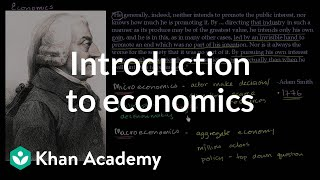Introduction to economics | Supply, demand, and market equilibrium | Economics | Khan Academy

Learn about some of the key ideas that influenced early economic thinkers, such as Adam Smith, in this video. Practice this yourself on Khan Academy right now: https://www.khanacademy.org/economics-finance-domain/macroeconomics/macro-basic-economics-concepts/macro-scarcity/e/introduction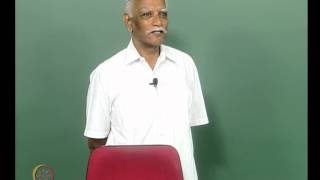Mod-01 Lec-33 Evolutionary Economics: the idea of change as evolution

History of Economic Theory by Dr. Shivakumar, Department of Humanities and Social Sciences IIT Madras, For more details on NPTEL visit http://nptel.iitm.ac.in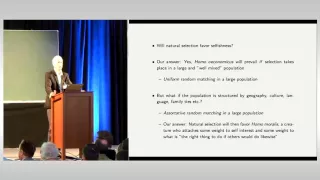Panorama of Mathematics: Jörgen W. Weibull

Panorama of Mathematics To celebrate the tenth year of successful progression of our cluster of excellence we organized the conference "Panorama of Mathematics" from October 21-23, 2015. It outlined new trends, results, and challenges in mathematical sciences. Jörgen W. Weibull: "Evoluti

From playlist Panorama of Mathematics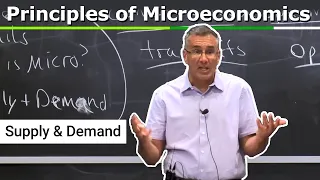1. Introduction and Supply & Demand

MIT 14.01 Principles of Microeconomics, Fall 2018 Instructor: Prof. Jonathan Gruber View the complete course: https://ocw.mit.edu/14-01F18 YouTube Playlist: https://www.youtube.com/playlist?list=PLUl4u3cNGP62oJSoqb4Rf-vZMGUBe59G- In this video, Prof. Gruber discusses the details of the co

From playlist MIT 14.01 Principles of Microeconomics, Fall 2018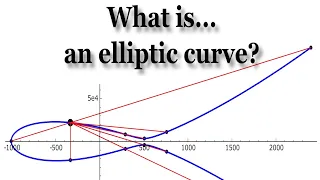What is... an elliptic curve?

In this talk, we will define elliptic curves and, more importantly, we will try to motivate why they are central to modern number theory. Elliptic curves are ubiquitous not only in number theory, but also in algebraic geometry, complex analysis, cryptography, physics, and beyond. They were

From playlist An Introduction to the Arithmetic of Elliptic CurvesM3 Challenge Final Event Award Ceremony 2016

Watch the first, second, third place teams and finalists as they're named during the 2016 Final Event Awards Ceremony! In the 2016 Moody's Mega Math Challenge, more than 5,000 high school students from across the U.S. used mathematical modeling to determine the best solutions for issues fa

From playlist M3 Challenge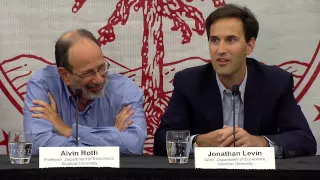Al Roth: 2012 Nobel Laureate in Economic Science Press Conference

October 15, 2012 - Economist Al Roth has been awarded the Sveriges Riksbank Prize in Economic Sciences in Memory of Alfred Nobel for his work on market design. He shares the prize with Lloyd Shapley, professor emeritus at UCLA. Roth, a visiting professor who will become a full Stanford fac

From playlist Stanford 2012 Nobel Prize Winners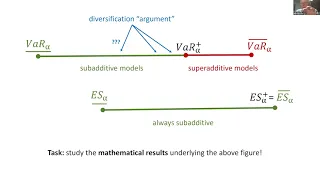Dependence Uncertainty and Risk - Prof. Paul Embrechts

Abstract I will frame this talk in the context of what I refer to as the First and Second Fundamental Theorem of Quantitative Risk Management (1&2-FTQRM). An alternative subtitle for 1-FTQRM would be "Mathematical Utopia", for 2-FTQRM it would be "Wall Street Reality". I will mainly conce

From playlist Uncertainty and Risk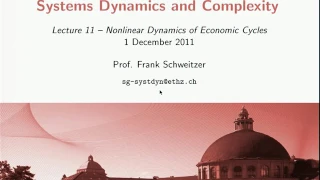ETH Lecture 11. Nonlinear Dynamics of Economic Cycles (01/12/2011)

Course: Systems Dynamics and Complexity (Fall 2011) from ETH Zurich. Source: http://www.video.ethz.ch/lectures/d-mtec/2011/autumn/351-0541-00L.html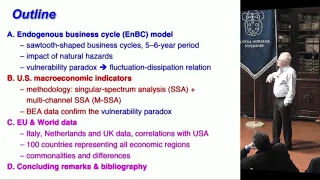Michael Ghil, Coupled Climate–Economics Modeling and Data Analysis - 21 January 2019

Coupled Climate–Economics Modeling and Data Analysis: Endogenous Business Cycles and Fluctuation–Dissipation Theory Michael Ghil Ecole Normale Supérieure, Paris, e University of California, Los Angeles Abstract: This talk will address the mathematics of non-equilibrium macroeconomics [

From playlist Centro di Ricerca Matematica Ennio De Giorgi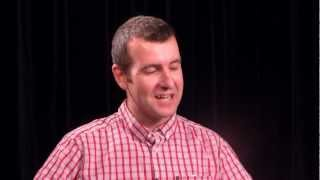Mathematician and DJ: Chris Tisdell interview

An interview with UNSW mathematician Dr Chris Tisdell. He discusses his relationship with mathematics and DJing.

From playlist DJ Chris TisdellNumber theory Full Course [A to Z]

Number theory (or arithmetic or higher arithmetic in older usage) is a branch of pure #mathematics devoted primarily to the study of the integers and integer-valued functions. Number theorists study prime numbers as well as the properties of objects made out of integers (for example, ratio

From playlist Number Theory